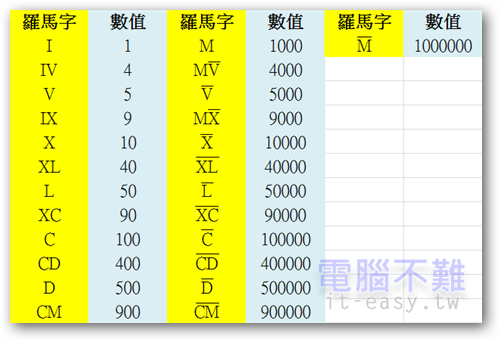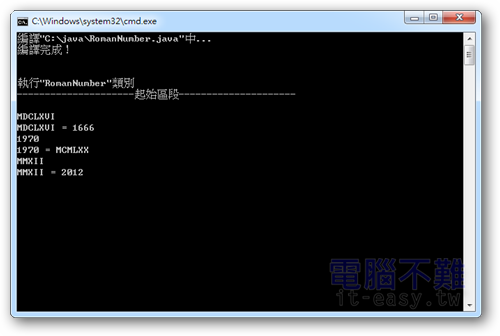# [Java]阿拉伯數字與羅馬數字轉換程式```1 2 3 4 5 6 7 8 9 10 11 12 13 14 15 16 17 18 19 20 21 22 23 24 25 26 27 28 29 30 31 32 33 34 35 36 37 38 39 40 41 42 43 44 45 46 47 48 49 50 51 52 53 54 55 56 57 58 59 60 61 62 63 64 65 66 67 68 69 70 71 72 73 //羅馬數字轉換程式   import java.util.Scanner; //引入Scanner工具   public class RomanNumber{ private final static String rnums[]={"m","cm","d","cd","c","xc","l","xl","x","Mx","v","Mv","M","CM","D","CD","C","XC","L","XL","X","IX","V","IV","I"}; //儲存所有羅馬數字 private final static int anums[]={1000000,900000,500000,400000,100000,90000,50000,40000,10000,9000,5000,4000,1000,900,500,400,100,90,50,40,10,9,5,4,1}; //儲存羅馬數字表示的值   public static void main(String[] args){ //程式進入點 Scanner sc=new Scanner(System.in); //建立Scanner物件 String input; //宣告input字串，用來儲存使用者輸入的值 while(sc.hasNext()){ //若還有資料尚未讀取，則執行 do{ input=sc.nextLine(); //讀取一行字串 }while(input.trim().length()<1); //如果沒有輸入任何字串，就重新讀取 if(input.charAt(0)=='#'){ //輸入井字號離開 break; } if(isDigit(input)){ //如果是整數 int n = Integer.parseInt(input); //將字串轉為整數 if(n>0&&n<4000000){ //如果n大於0且小於4000000 System.out.println(input + " = " + toRnums(n)); }else{ //如果超過範圍 System.out.println("輸入的阿拉伯數字有誤！必須大於零且小於四百萬！"); } }else{ //如果不是整數 int n = toAnums(input); //將羅馬字串轉為阿拉伯數字(數值) if(toRnums(n).equals(input)) { //判斷輸入的羅馬字串格式是否正確(轉成阿拉伯數字(數值)後再轉回羅馬字串，並與原本的字串做比較) System.out.println(input + " = " + n); }else{ System.out.println("輸入的羅馬數字有誤！"); } } } } public static boolean isDigit(String s){ //判斷是否為整數 try { int i = Integer.parseInt(s); //將字串轉為整數 } catch(Exception e) { //如果轉換失敗，會丟出例外 return false; //不是整數 } return true; //是整數 } public static String toRnums(int num){ //將整數轉成以字串表示的羅馬數字 if(num==0){ //因為羅馬數字裡並沒有零，所以輸出ZERO！ return "ZERO"; }else if(num<0||num>3999999){ //溢位判斷 return "OVERFLOW"; }   StringBuilder output = new StringBuilder(); //儲存羅馬數字字串 for(int i=0;num>0&&i<anums.length;i++) //尋找對應的羅馬數字 { while(num>=anums[i]){ //將羅馬數字加到output物件內 num-=anums[i]; output.append(rnums[i]); } } return output.toString(); //傳回羅馬數字字串 } public static int toAnums(String s){ //將羅馬數字轉成阿拉伯數字(數值) int num=0; StringBuilder Buffer = new StringBuilder(s); //將字串s存入字串緩衝區內 for(int i=0;Buffer.length()>0&&i<anums.length;i++) //尋找對應的羅馬數字 { while(Buffer.indexOf(rnums[i])==0){ //如果有找到相同的羅馬數字字串，且位置在字串最左邊 num+=anums[i]; //將羅馬數字轉為阿拉伯數字(數值)並存到num變數內 Buffer.delete(0,rnums[i].length()); //刪除已讀取到的字串 } } return num; //傳回轉換後的阿拉伯數字(數值) } }```